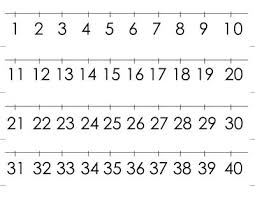# The Roman

The Roman numerals are read from left to right and they use an additive system (such as is the case in VII = 7) and subtractive system (such as is the case in IX = 9). How would you explain to your learners how they should go about deciphering the following Roman numerals: MMXIX? Also, give the answer.

x =  2019

### Step-by-step explanation:Did you find an error or inaccuracy? Feel free to write us. Thank you!

Matik

Matik
Compare the Egyptian and the Babylonian numeration systems in terms of the number of symbols, functionality and possible uses by answering the questions below:
a) Write down the similarities and differences between the two systems.
b) Are these numeration systems still used today? Do your own research and then try to give reasons with references for your answer.
c) Do we still use the Roman numeration system today? If so, give examples from your own environment.

1 month ago  3 Likes